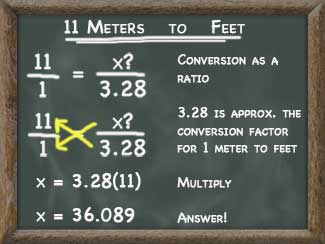# Kyle's Converter

### Unit Conversions Basics -- How to convert anythingAlmost all conversions can be solved using a simple ratio.

In the case of units measuring things like distance, speed, or mass the zero position is set at fixed locations, e.g. objects having no length, at rest, or having no mass. Units having a fixed zero position like these are said to use a ratio number scale because a unit having a value of 10 is twice as large as a unit having a value of 5.

Conversion can be expressed as a ratio and calculated by knowing the conversion factor acquired ahead of time. Conversion factors can be determined by measurement or mathematically if units have some common origin.

Temperature measurement is the major exception to the ratio number scale. The zero position on the Celsius, Fahrenheit, and most other temperature scales is set based on what the designer of the scale thought was logical. Therefore a temperature of 10°C is not twice as hot as a temperature of 5°C. These types of conversions exist on an interval scale and can be handled by using a ratio to resize the units and then adjusted the zero position as required.

The tools provided above should solve most of your ratio (and some interval) conversion needs and feel free to share them too!

#### How to find the conversions you're looking for:

1. Conversions are organized into several upper level categories.
2. All units within a given category can be converted to any other unit within that category. First choose the unit you want to convert from:
3. Then select the link for the unit you want to convert it to.
4. Calculate and Share!